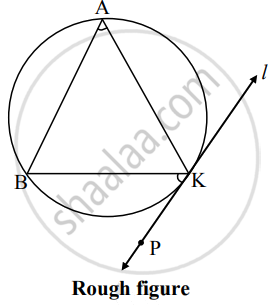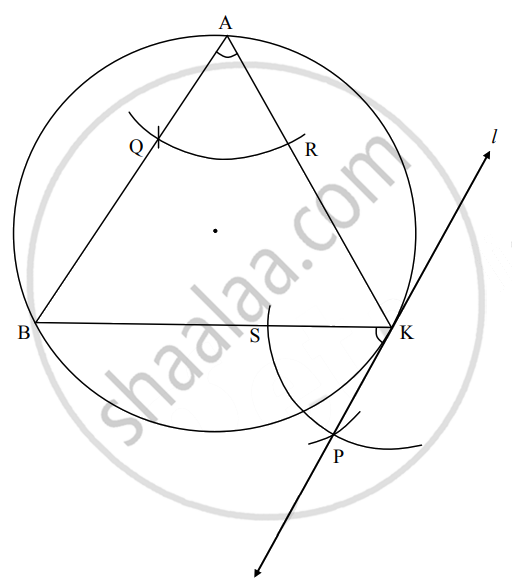# Draw a circle with a radius of 3.5 cm. Take the point K anywhere on the circle. Draw a tangent to the circle from K (without using the center of the circle) - Geometry

Diagram

Draw a circle with a radius of 3.5 cm. Take the point K anywhere on the circle. Draw a tangent to the circle from K (without using the center of the circle)

#### SolutionSteps of construction:

1. Draw a circle of radius 3.5 cm and take any point K on it.
2. Draw chord BK of any length and an inscribed ∠BAK of any measure.
3. By taking A as centre and any convenient distance on compass draw an arc intersecting the arms of ∠BAK in points Q and R.
4. With K as centre and the same distance in the compass, draw an arc intersecting the chord BK at point S.
5. Taking radius equal to QR and S as centre, draw an arc intersecting the previously drawn arc. Name the point of intersection as P.
6. Draw line KP.
Line KP is the required tangent to the circle.
Concept: Construction of a Tangent to the Circle at a Point on the Circle
Is there an error in this question or solution?

Share